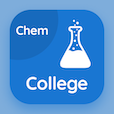Colleges Online Courses

College Chemistry MCQs

College Chemistry MCQ PDF - Topics

# Empirical Formula MCQ Quiz Online

Learn Empirical Formula Multiple Choice Questions (MCQ), Empirical Formula quiz answers PDF to study college chemistry online course for college chemistry classes. Basic Chemistry Multiple Choice Questions and Answers (MCQs), Empirical Formula quiz questions for 2 year online degrees. "Empirical Formula MCQ" PDF Book: spectrometer, empirical formula, relative abundance test prep for online schools that offer certificate programs.

"The empirical formula of benzene is similar to" MCQ PDF: empirical formula with choices water, glycerin, hydrogen peroxide, and glucose for 2 year online degrees. Study empirical formula quiz questions for merit scholarship test and certificate programs for best two year degrees.

## MCQs on Empirical Formula Quiz

MCQ: The empirical formula of benzene is similar to

water
glycerin
hydrogen peroxide
glucose

MCQ: The formula showing the simplest whole-number ratio is

scientific
mathematical
molecular
empirical

### More Topics from College Chemistry Course